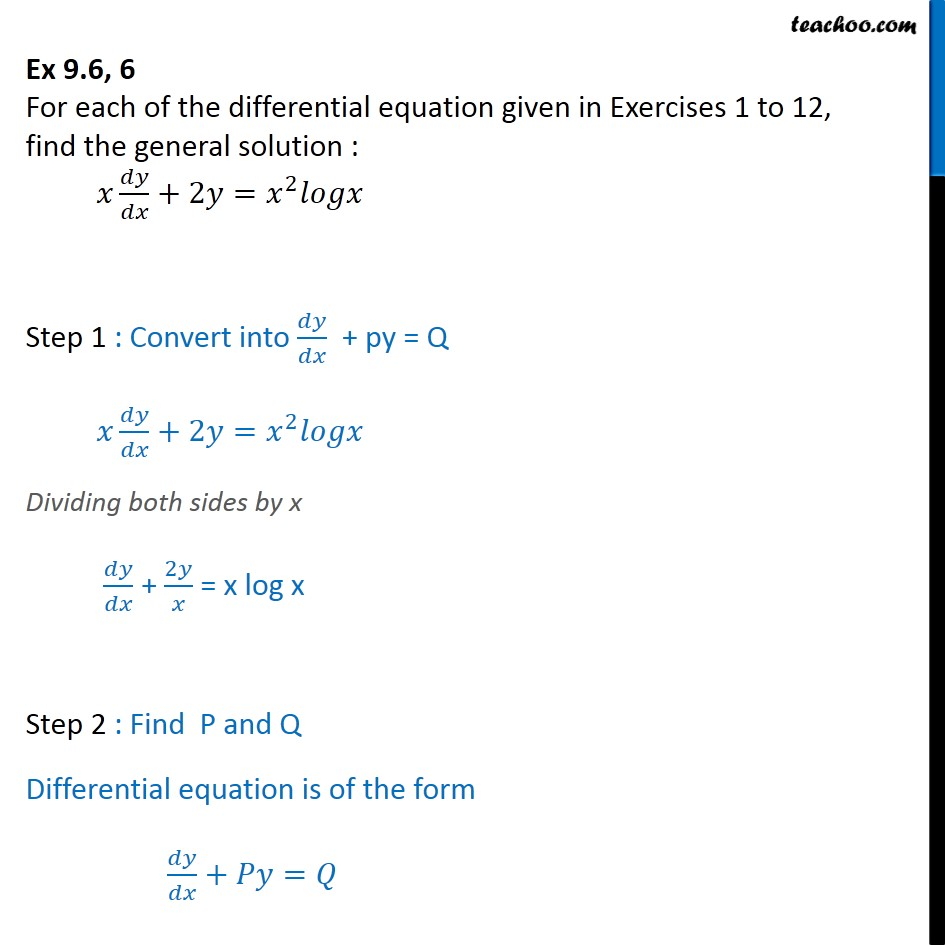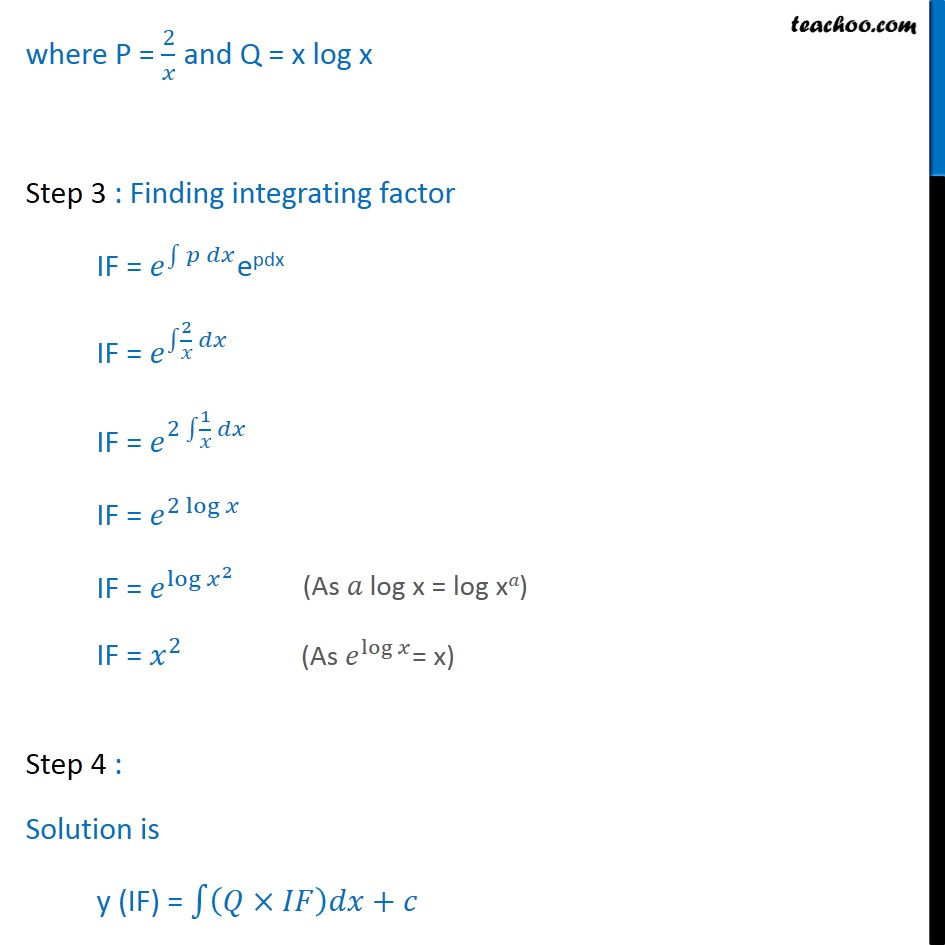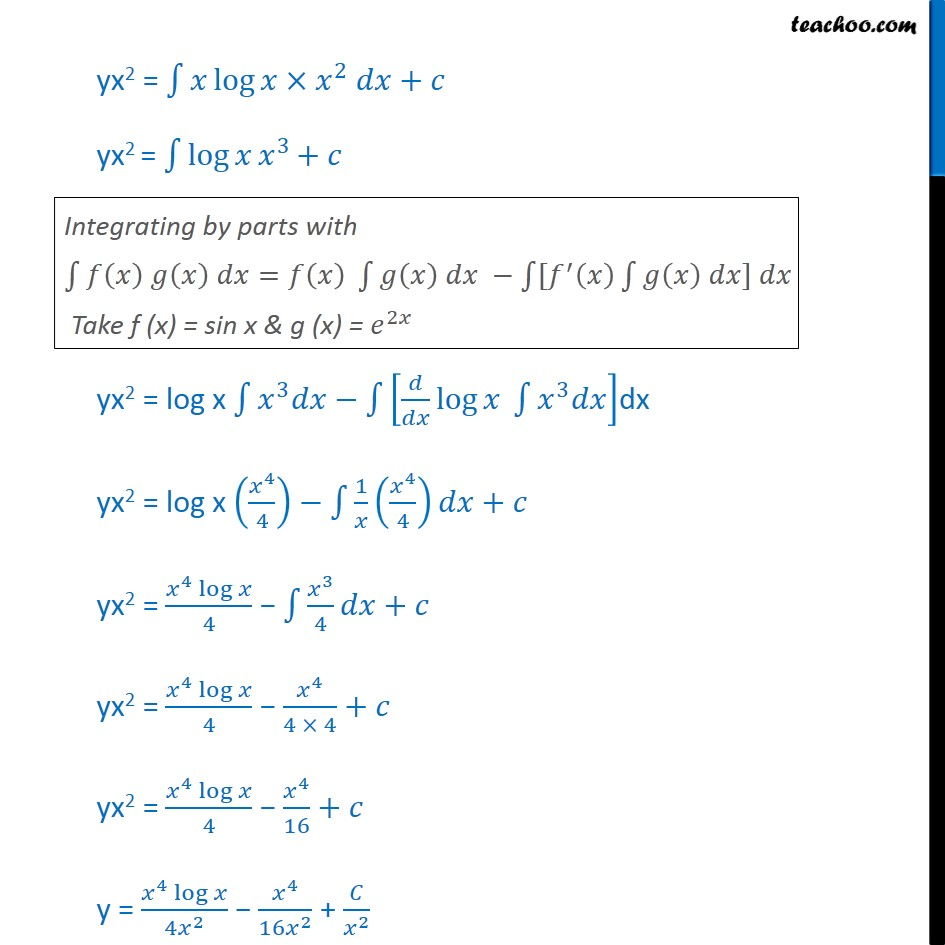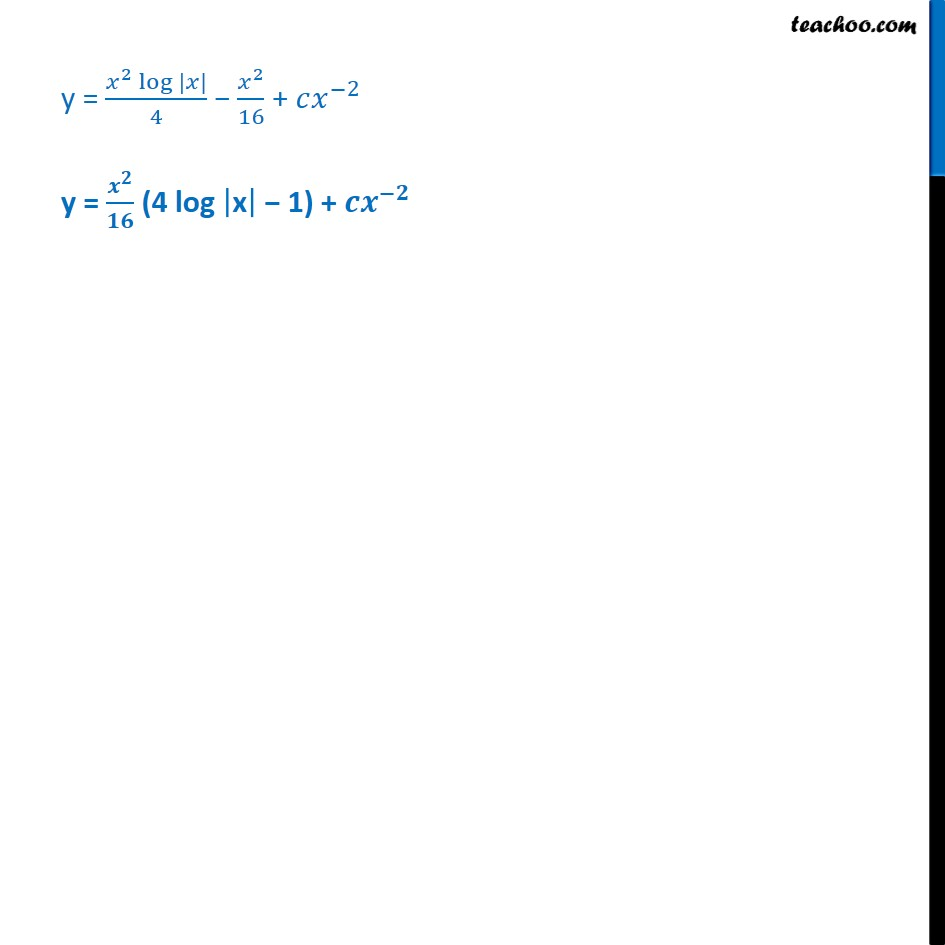1. Chapter 9 Class 12 Differential Equations
2. Serial order wise
3. Ex 9.6

Transcript

Ex 9.6, 6 For each of the differential equation given in Exercises 1 to 12, find the general solution : +2 = 2 Step 1 : Convert into + py = Q +2 = 2 Dividing both sides by x + 2 = x log x Step 2 : Find P and Q Differential equation is of the form + = where P = 2 and Q = x log x Step 3 : Finding integrating factor IF = epdx IF = 2 IF = 2 1 IF = 2 log IF = log 2 IF = 2 Step 4 : Solution is y (IF) = + yx2 = log 2 + yx2 = log 3 + yx2 = log x 3 log 3 dx yx2 = log x 4 4 1 4 4 + yx2 = 4 log 4 3 4 + yx2 = 4 log 4 4 4 4 + yx2 = 4 log 4 4 16 + y = 4 log 4 2 4 16 2 + 2 y = 2 log | | 4 2 16 + 2 y = (4 log x 1) +

Ex 9.6